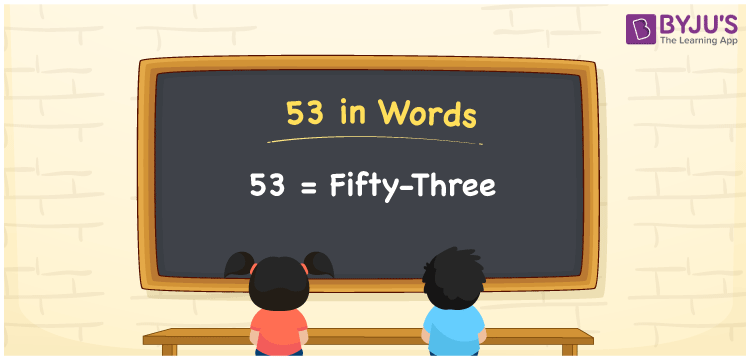# 53 in Words

53 in words is written as “Fifty-three”. For example, Shyam bought a Pencil box of Rupees Fifty-three. In Mathematics, 53 is a natural number that is used to represent a value or a quantity. Learn to read and write numbers in words at BYJU’S, using the place value chart.

 53 in Words Fifty-three Fifty-three in Numbers 53

## 53 in English Words## How to Write 53 in Words?

53 is expressed as Fifty-three in words. To read and write 53 in words, we can use here place value method. Since 53 is a two-digit number, thus;

 Tens Ones 5 3

From the above table, we can see, 5 is at tens place (50) and 3 is at ones place. We need to read the number from right to left, in the table. Thus, 50 + 3 = 53, together we can spell the number as Fifty-three.

### Expanded Form of 53

We can write the expanded form of 53 as:

5 at Tens place + 3 at Ones place

= 5 × 10 + 3 × 1

= 53

= Fifty-three

53 is a whole number that is succeeded by 52 and preceded by 54. Find more about the number 53 below:

• 53 in Words – Fifty-three
• Is 53 an odd number? – Yes
• Is 53 an even number? – No
• Is 53 a perfect square number? – No
• Is 53 a perfect cube number? – No
• Is 53 a prime number? – Yes
• Is 53 a composite number? – No

## Frequently Asked Questions on 53 in words

Q1

### What is 53 in words?

53 in words is given by Fifty-three.
Q2

### What is the rule to write 53 in words?

53 in words is written based on the place values of each digit. 5 is at tens place and 3 is at ones place, so together we can write as Fifty-three.
Q3

### What is the place value of 5 in 53?

The place value of 5 in 53 is Tens.